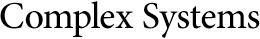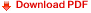## Neural Control Model for an Inverted Double PendulumAlexander M. Coxe

#### Abstract

Rolling cart stabilization for the inverted double pendulum in analog to the usual system of the inverted pendulum is explored. An appropriate linearization for the equations of motion near equilibrium is noted, and a proportional derivative controller is developed. This system is run from many initial conditions through many time steps to generate multiple time series of algorithm response to the imbalanced system. These time series are repurposed as a list of associations and used as a large training set for an artificial neural network. When applied to the inverted double pendulum system, the trained neural network is seen to provide much more stable control than the algorithm from which it was trained. It appears the neural network learns predictive stabilization from the training set and is able to react to the system more quickly than the algorithm can compute a correction.

Keywords: neural networks; control systems, optimal control; computational irreducibility